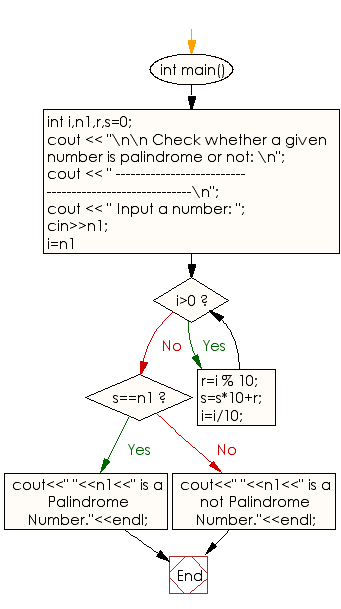﻿ C++ : Check whether a given number is palindrome or not

# C++ Exercises: Check whether a given number is palindrome or not

## C++ Numbers: Exercise-38 with Solution

Write a C++ program to check whether a given number is a palindrome or not.

Sample Solution:

C++ Code :

``````#include <iostream>
using namespace std;
int main()
{
int i,n1,r,s=0;
cout << "\n\n Check whether a given number is palindrome or not: \n";
cout << " -------------------------------------------------------\n";
cout << " Input a number: ";
cin>>n1;
for(i=n1;i>0; )
{
r=i % 10;
s=s*10+r;
i=i/10;
}
if(s==n1)
{
cout<<" "<<n1<<" is a Palindrome Number."<<endl;
}
else
{
cout<<" "<<n1<<" is a not Palindrome Number."<<endl;
}
}
``````

Sample Output:

```Check whether a given number is palindrome or not:
-------------------------------------------------------
Input a number: 141
141 is a Palindrome Number.
```

Flowchart:C++ Code Editor: